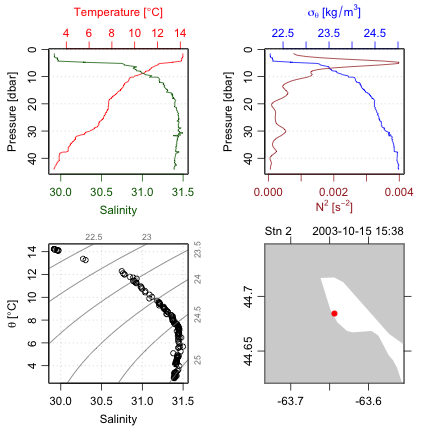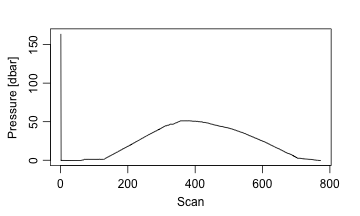# 2. Analysis of CTD data

#### 2022-07-05

Abstract. The basics of working with CTD data are outlined in this vignette, as a supplement to a shorter entry in the main vignette.

# 1 Example with pre-trimmed data

Many of the object types supported by oce come with built-in data. For an example, data(ctd) yields a CTD profile that has been trimmed to just the downcast portion of the sampling. (See the next section to learn how to do this trimming.) A summary and plot (Figure 2) are created as follows.

library(oce)
data(ctd)
summary(ctd)
#> CTD Summary
#> -----------
#>
#> * Instrument:          SBE 25
#> * Temp. serial no.:    1140
#> * Cond. serial no.:    832
#> * File:                "/Users/kelley/git/oce/create_data/ctd/ctd.cnv"
#> * Original file:       c:\seasoft3\basin\bed0302.hex
#> * Start time:          2003-10-15 15:38:38
#> * Sample interval:     1 s
#> * Cruise:              Halifax Harbour
#> * Vessel:              Divcom3
#> * Station:             Stn 2
#> * Location:            44.684N 63.644W
#> * Data Overview
#>
#>                                 Min.   Mean   Max. Dim. NAs OriginalName
#>     scan                         130    220    310  181   0         scan
#>     timeS [s]                    129    219    309  181   0        timeS
#>     pressure [dbar]             1.48 22.885 44.141  181   0           pr
#>     depth [m]                  1.468 22.698 43.778  181   0         depS
#>     temperature [°C, IPTS-68]  2.919 7.5063 14.237  181   0         t068
#>     salinity [PSS-78]         29.916 31.219 31.498  181   0        sal00
#>     flag                           0      0      0  181   0         flag
#>
#> * Processing Log
#>
#>     - 2018-11-14 20:03:47 UTC: create 'ctd' object
#>     - 2018-11-14 20:03:47 UTC: read.ctd.sbe(file = file, debug = 10, processingLog = processingLog)
#>     - 2018-11-14 20:03:47 UTC: oce.edit(x = ctd, item = "startTime", value = as.POSIXct(gsub("1903",     "2003", format(ctd[["startTime"]])), tz = "UTC") + 4 * 3600,     reason = "file had year=1903, instead of 2003", person = "Dan Kelley")
plot(ctd)Figure 2. An overview of a ctd dataset.

Accessing the data within this ctd object can be done directly, e.g. ctd@data\$pressure holds the pressure record, but it is usually better to use an accessor function that is provided with oce. This function is named [[, and it takes a character string as an argument, e.g. ctd[["pressure"]] yields the pressure column. The accessor notation is preferable to direct access because it is simpler for the user. For example, several oce objects store the data in single-byte or two-byte chunks, to match the raw format used by the instruments, and the accessor function takes care of translating these values to what are sometimes called “science” units.

Exercise 1. Plot a profile of $$\sigma_\theta$$ and $$N^2$$ within the pycnocline in the built-in ctd data set. (Hint: use subset().)

# 2 Example with raw data

Practicing Oceanographers may be wondering how the CTD cast used in the preceding section was trimmed of equilibration-phase and upcast-phase data. Spurious data from these phases must be trimmed as a first step in processing. For example, consider the following code.

data(ctdRaw)
plotScan(ctdRaw)Figure 3. Scanwise plot of the ctdRaw sample data set. Note the spike at the start, the equilibration phase before the downcast, and the spurious freshening signal near the start of the upcast.

This produces a two-panel plot (Figure 3) of the data as a time-series, revealing not just the desired downcast, but also an earlier equilibration phase and a later upcast. The x-axis in Figure 3 is the scan number, which is a convenient index for extraction of the downcast portion of the profile by an essentially manual method, e.g. proceeding with a sequence of commands such as

plotScan(ctdTrim(ctdRaw, "range",
parameters=list(item="scan", from=140, to=250)))
plotScan(ctdTrim(ctdRaw, "range",
parameters=list(item="scan", from=150, to=250)))

This method of making decisions based on plotted information is probably the most robust method of trimming data. However, for quick work, users may be satisfied with the results of automatic downcast detection, e.g.

ctdTrimmed <- ctdTrim(ctdRaw)

It should be noted that ctdTrim() inserts entries into the object’s log file, so that the details of how the trimming was done are recorded together with the data.

Once the profile has been trimmed, one may wish to use ctd.decimate() to smooth the data and interpolate the smoothed results to uniformly-spaced pressure values.

Taking these things together, a quick visual examination of a CTD file takes just one line of code:

plot(ctdDecimate(ctdTrim(read.ctd("stn123.cnv"))))

# 3 Example with WOCE archive data

The package has a harder time scanning the headers of data files in the WOCE archive format than it does in the Seabird format illustrated in the previous examples. This is mainly because front-line researchers tend to work in the Seabird format, and partly because the WOCE format is odd. For example, the first line of a WOCE file is of the form CTD,20060609WHPOSIODAM (or BOTTLE,...). Scanning the item to the left of the comma is not difficult (although there are variants to the two shown, e.g. CTDO sometimes occurs). The part to the right of the comma is more difficult. The first part is a date (yyyymmdd) so that is no problem. But then things start to get tricky. In the example provided, this text contains the division of the institute (WHPO), the institute itself (SIO), and initial of the investigator (DAM). The problem is that no dividers separate these items, and that there seem to be no standards for the item lengths. Rather than spend a great deal of time coding special cases (e.g. scanning to see if the string WHOI occurs in the header line), the approach taken with oce is to ignore such issues relating to quirky headers, on the assumption that users can scan human-written headers with high skill.

Quite commonly, CTD profiles taken during a cruise are collected together in a sequence of files in a given directory. For a real-world example, one might visit the website mentioned in the code provided below, download and expand the zip file, enter the directory thus formed, and run the code to get an overall TS plot for all the CTD stations of this cruise. (Caution: the link seems to change from time to time.)

library(oce)
# http://cchdo.ucsd.edu/data/7971/ar18_58JH19941029_ct1.zip
files <- system("ls *.csv", intern=TRUE)
for (i in 1:length(files)) {
if (i == 1) {
plotTS(x, Slim=c(31, 35.5), Tlim=c(-2, 10), type='o')
} else {
points(x[["salinity"]], x[["potential temperature"]])
lines(x[["salinity"]], x[["potential temperature"]])
}
}

The [[ notation is explained in Section 3, but this example conveys the gist, that it permits accessing data, or derived data, from within an object.

In the above, lines connect the points within a given profile. This can be a useful method for a quick scan looking for outliers. Another is to colour-code the profiles, although this gets confusing with large datasets, in which case the method of the following exercise might be useful.

Exercise 2. (advanced) Make a multi-file plot summarizing the TS relationship in a collection of CTD datasets, with each plot file showing the overall relationship in gray and the individual profile in black.

# 4 Solutions to exercises

Exercise 1. Plot a profile of $$\sigma_\theta$$ and $$N^2$$ within the pycnocline in the built-in ctd data set. (Hint: use subset().)

Although one may argue as to the limits of the pycnocline, for illustration let us say it is in 5 dbar to 12 dbar range. One way to do this is

library(oce)
data(ctd)
pycnocline <- ctdTrim(ctd, "range",
parameters=list(item="pressure", from=5, to=12))
plotProfile(pycnocline, which="density+N2")

Another is

library(oce)
data(ctd)
pycnocline <- subset(ctd, 5 <= pressure & pressure <= 12)
plotProfile(pycnocline, which="density+N2")

Exercise 2. (advanced) Make a multi-file plot summarizing the TS relationship in a collection of CTD datasets, with each plot file showing the overall relationship in gray and the individual profile in black.

The code provided below creates 91 PNG files, with names ar18_01.png, ar18_02.png, etc. Loading these in a view that permits quick paging through this file list is an easy way to spot suspicious data, since each plot has the station number at the top. (Users trying this example should bear in mind that this is a fairly large dataset, so the processing will take up to a minute.)

library(oce)
# http://cchdo.ucsd.edu/data/7971/ar18_58JH19941029_ct1.zip
files <- system("ls *.csv", intern=TRUE)
n <- length(files)
ctds <- vector("list", n) # to hold the CTD objects
station <- vector("list", n)
for (i in 1:n) {
dev.off()